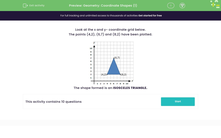# Geometry: Coordinate Shapes (1)

In this worksheet, students plot points on xy axes and identify the name of the shape formed.Key stage:  KS 2

Curriculum topic:   Geometry: Position and Direction

Curriculum subtopic:   Know, Describe and Present Shapes

Popular topics:   Geometry worksheets

Difficulty level:#### Worksheet Overview

Look at the x and y- coordinate grid below.

The points (4,2), (6,7) and (8,2) have been plotted.

When we plot coordinates, remember the first number is for the x-axis and the second number is for the y-axis.

The x-axis is the horizontal line at the bottom and the y-axis is the vertical line going up the left-hand side but, don't worry, they're labelled for you!The shape formed is an ISOSCELES TRIANGLE.

An isosceles triangle is a triangle that has two of its sides and two of its angles the same.

An equilateral triangle is a regular shape - this means it has all of its sides and angles the same size.

A scalene triangle is a triangle where all three angles are different and all three sides are different.

A right-angled triangle has a right-angle in it - this is when two of its sides meet at a 90 degree angle.

### What is EdPlace?

We're your National Curriculum aligned online education content provider helping each child succeed in English, maths and science from year 1 to GCSE. With an EdPlace account you’ll be able to track and measure progress, helping each child achieve their best. We build confidence and attainment by personalising each child’s learning at a level that suits them.

Get started